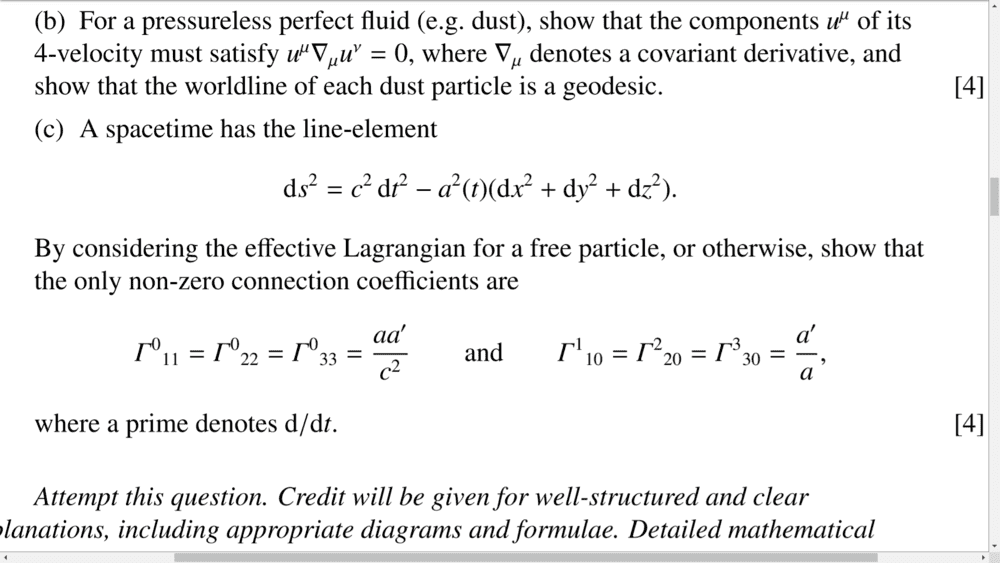# General Relativity easy question

Homework Statement:
Connection coefficients
Relevant Equations:
part c) of the problemsAttempt: I don't know what they mean by effective Lagrangian.
I am aware there is something called 'the lagrangian' that goes as L=g_ab * dx^a/dk * dx^b/dk, but i dont see how this gives me any of the chrostoffel symbols...

cheers

## Answers and Replies

Well, there are two ways of getting the connection coefficients.
One way is getting them via the formula where they are defined as Christoffel symbols of the second kind:
$$\Gamma^\mu_{\nu\rho} = \frac{1}{2}g^{\mu\sigma}(\partial_\nu g_{\sigma\rho} + \partial_\rho g_{\nu\sigma} - \partial_\sigma g_{\nu\rho})$$
The second way is by variation of action:
$$I = \frac{1}{2}\int g_{\mu\nu}\dot{x^\mu}\dot{x^\nu}d\lambda$$
The Lagrangian in this action is so called geodesic Lagrangian, and by variating the action ##\delta I = 0##, you find geodesic equations from which you can read connection coefficients. You variate with respect to every component ##x^\mu##. Since in general relativity, free particles travel along geodesics, this is what is meant by effective Lagrangian for free particles(I think, at least, I always called it geodesic Lagrangian, but there should be no other). Of course, ##\dot{x^\mu} \equiv \frac{dx^\mu}{d\lambda}## where ##\lambda## is parameter of the geodesic.
Sometimes the action is also defined as:
$$I = \int \sqrt{g_{\mu\nu}\dot{x^\mu}\dot{x^\nu}}d\lambda$$
but these two actions have same equations of motion. The second action is precisely the line element form, but it's pretty much the same, and I like using the one without the square root.

•DEvens
DEvens
Education Advisor
Gold Member